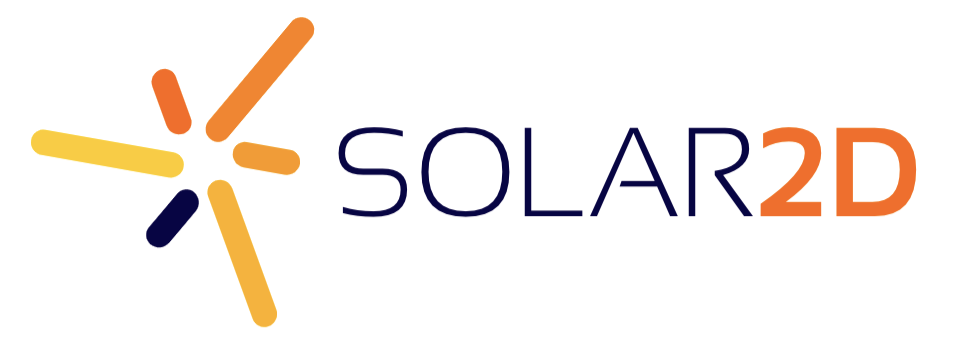# Full 360 Rotation Character Movement

Hello, I’m currently using the default player movement that is included with MTE. However, the default movement is only a tile by tile movement, and I would like full 360 movement, not grid locked.

If anyone has any sample code or could provide a way to do that, I’d appreciate it!

Here is the current player movement code

``-- DETECT MOVEMENT ------------------------------------------------------------ local movement = nil local function move( event ) if event.phase == "began" then display.getCurrentStage():setFocus(event.target, event.id) event.target.isFocus = true end if event.phase == "began" or event.phase == "moved" then local dirX = event.x - event.target.x local dirY = event.y - event.target.y local angle = math.deg(math.atan(dirY/dirX)) if dirX \< 0 then angle = 90 + (90 - (angle \* -1)) end angle = angle + 90 angle = math.round(angle / 90) \* 90 if angle == 360 then angle = 0 end if angle % 90 ~= 0 then movement = nil else movement = tostring(angle) end elseif event.phase == "ended" or event.phase == "cancelled" then movement = nil display.getCurrentStage():setFocus( event.target, nil ) event.target.isFocus = false end return true end``